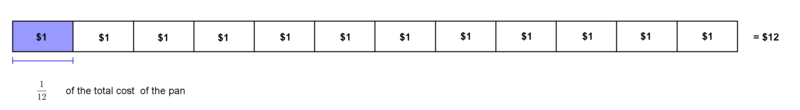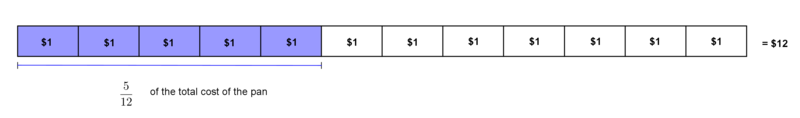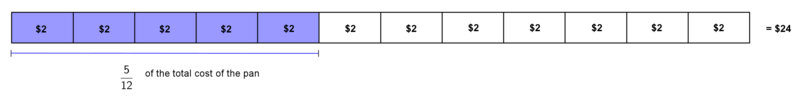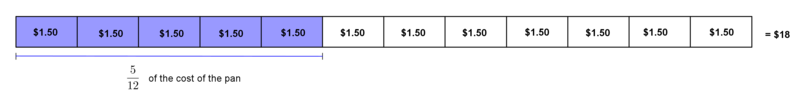##### Engage your students with effective distance learning resources. ACCESS RESOURCES>>

Alignments to Content Standards: 5.NF.B.4

The 5th graders want to raise money for their overnight camping trip by selling cornbread during the school Chili Cook-Off contest. All the pans of cornbread are square.  A pan of cornbread costs $\12$. The customers can buy any fractional part of a pan of cornbread and pay that fraction of $\12$. For example, $\frac{1}{2}$ of a full pan costs $\frac{1}{2}$ of $\12$.

1. Mrs. Farmer buys cornbread from a pan that is $\frac{1}{4}$ full.  She buys $\frac{1}{3}$ of the remaining cornbread in the pan.
• What fraction of the whole pan of cornbread does she buy?  Use objects and/or a diagram to show how much of the pan of cornbread she buys.

• What does she pay for the cornbread she bought?  Use objects and/or a diagram to show how much pays.

1. The next customer is the school principal.  He buys cornbread from a different pan that is $\frac{1}{2}$ full. He buys $\frac{5}{6}$ of the remaining cornbread in the pan.
• What fraction of the whole pan of cornbread does he buy?  Use a diagram to show how much of the pan of cornbread he  buys.

• What does he pay for the cornbread he bought?  Use a diagram to show how much he will pay for his part of the pan.

1. What would be the cost of the cornbread the principal bought if the price of the entire pan changed to:

• $\24$

• $\6$

• $\18$

Use a diagram to explain each answer.

## IM Commentary

This task is designed to introduce students to an area representation for multiplying a fraction by a fraction.  Note: A number line or other representation could be used; but if this is used at the beginning of a unit, students will likely try to draw a literal representation of the situation.  Students should be provided an object to represent the pan of cornbread (e.g. a Post-It note) that can be folded or drawn on to show the fractions in the situation.  The hands-on manipulative will allow the students to explore this new concept of a part of a part concretely.  It may also help to have manipulative money available as a concrete model for students to use while solving.  For another layer of complexity, the teacher can introduce dimensions of the pan--1 ft x 1 ft.  This gives a deeper meaning to the fractions used within the context of the problem.  For example, $\frac{1}{4}$ of the pan means that piece of cornbread will be $\frac{1}{4}$ foot long on one side. This is true because the pan is square, and it only works if you cut the cornbread in one direction for one fraction and then perpendicular to those cuts for the other fraction. Depending on your students and whether this is an introductory lesson, you may want to explore this concept in depth through class discussion.

Part 1—Students are asked to start by finding a fraction of a fraction.  Using two different colored pencils will help the students record their thoughts on paper as they draw a diagram.  The unit fractions are intentionally used at the beginning to help the students see that that the pan is actually cut into twelfths for Part 1.  They may try to report a solution that does not take into account that the third is cut into fourths which are then extended into the entire pan to create twelfths (see solution below.)  There should be class discussion on the operation that is occurring.  Many students will not intuitively understand that this is a multiplication problem instead of division.  Then students are asked to multiply a fraction by a whole number with the price of the pan.  This requires students to rethink what is the “whole” they are examining.  The whole now means the price of the entire pan or $\12$. This ability to switch between different “wholes” will help lay those foundational skills for proportional reasoning and ratios in the middle level grades.

Part 2—At this point, the problem evolves as unit fractions are left behind.  The price of the pan still corresponds to the twelfths of the pan nicely ($\frac{1}{12}=\1$). However, this part leads into Part 3.

Part 3—Students are now asked to change the cost of the pan while keeping the amount of the pan purchased the same.  This again requires the ability of students to change their concept of the whole they are examining.  All three of the new prices encourage students to make portions of a dollar in order to solve. A possible extension to part 3 could encourage students to look at the relationship between the original price and the proposed changes (it doubles, it is half, it is three halves as large).

This task was written as a part of a collaborative project between Illustrative Mathematics, the Smarter Balanced Digital Library, and the Teaching Channel.

## Solutions

Solution: A

1. Mrs. Farmer purchased $\frac{1}{12}$ of the pan of cornbread.1. $\frac1{12} \times 12 = 1$. Mrs. Farmer will pay $1 for her fraction of the pan.Solution: B 1. The principal purchased$\frac5{12}$of the whole pan.1.$\frac5{12} \times 12 = 5$. The principal will pay$5 for his fraction of the pan.Solution: C

1. The principal will pay $\frac5{12}$ of $\24$, which is $\10$1. The principal will pay $\frac5{12}$ of $\6$, which is $\2.50$.1. The principal will pay $\frac5{12}$ of $\18$, which is $\7.50$.